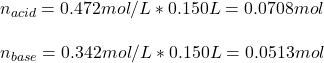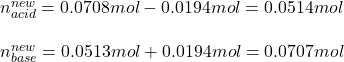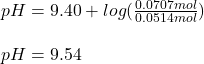## A buffer solution contains 0.472 M hydrocyanic acid and 0.342 M sodium cyanide. If 0.0194 moles of sodium hydroxide are added to 150 mL of t

Question

A buffer solution contains 0.472 M hydrocyanic acid and 0.342 M sodium cyanide. If 0.0194 moles of sodium hydroxide are added to 150 mL of this buffer, what is the pH of the resulting solution

in progress 0
6 months 2021-07-18T22:56:24+00:00 1 Answers 0 views 0

pH = 9.54.

Explanation:

Hello there!

In this case, since this is an acidic buffer due to the hydrocyanic acid, it will be firstly necessary for us to calculate the moles of both acid and conjugate base in 150 mL given their concentrations:Next, since the effect of adding NaOH, consume the acid and produce more base, we subtract 0.0194 moles from 0.0708 mol and add 0.0194 to 0.0513 mol for us to calculate the new moles:Thus, since the pKa of hydrocyanic acid is 9.40, we therefore use the Henderson-Hasselbach equation to calculate the pH of the resulting solution for the same volume:Which increase make sense since we added some strong base.

Regards!